<

## Taxonomy Trail

Hidden : 5/10/2005
Difficulty: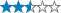Terrain:Size: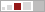(regular)

Join now to view geocache location details. It's free!

#### Watch

How Geocaching WorksPlease note Use of geocaching.com services is subject to the terms and conditions in our disclaimer.

### Geocache Description:

The above coordinates are for parking, which should be easy unless you’ve timed it to coincide with the school run, when it’s absolute bedlam

This is a multi-cache consisting of four micros and the final cache which is a medium sized plastic food box. Each micro will give you a clue to solve which will give you coordinates to the next micro and so on, until the final cache.

Unless you have a very good knowledge of botany you will have to take with you a book of British Wild Plants as each clue will give you the Common name of a wild flower. You will then have to find the Botanical name and the instructions below will give you the coordinates for the next cache. Depending on the time of year you might be able to see the plant mentioned in the clue. I’ve placed them where I have seen the flowers over the last couple of years. Your wild flower book should be a bit more concise than the Collins Gem Series!

An Ordnance Survey Map of the area would also be a good thing to take as all the caches are on public rights of way. Please keep to the paths and tracks. Some of the paths can be very muddy so wellies are a good idea. A short part of this cache is along a narrow country lane. Please take care.

The whole route is about 5 miles and follows a circular route from the parking at
N51’ 03.167 W001’ 10.158

The final cache contains the usual stuff but the first to find will get a voucher for 18 pints of beer from the local brewery (only given to people over the age of 18).

To start you off, look in your book for the current botanical name of the Lesser Celandine (It has changed relatively recently)

Micro 1
N 51’ 03.ABC
W001’11.DEF

A=THE NUMBER OF ‘F’s
B=THE NUMBER OF ‘N’s multiplied by 2
C=THE NUMBER OF ‘A’s multiplied by 2
D=THE NUMBER OF ‘I’s multiplied by 2
E=THE NUMBER OF ‘A’s multiplied by 3
F= THE NUMBER OF ‘R’s multiplied by 2

Micro2
N51’ 03.ABC
W001’ 12.DEF

A=THE NUMBER OF ’L’s
B=THE NUMBER OF ‘C’s multiplied by 4
C=THE NUMBER OF ‘S’s multiplied by 3
D=THE NUMBER OF ‘F’s
E=THE NUMBER OF ‘A’s multiplied by 4
F= THE NUMBER OF ‘R’s multiplied by 2

Micro3
N51’ 03.ABC
W001’ 10.DEF

A=THE NUMBER OF ’O’s multiplied by 3
B=THE NUMBER OF ‘B’s multiplied by 7
C=THE NUMBER OF ‘O’s
D=THE NUMBER OF ‘O’s multiplied by 3
E=THE NUMBER OF ‘R’s multiplied by 3
F= THE NUMBER OF ‘N’s X 2

Micro4
N51’ 03.ABC
W001’ 10.DEF

A=THE NUMBER OF ’E’s multiplied by 2 minus1
B=THE NUMBER OF ‘N’s multiplied by 3
C=THE NUMBER OF ‘R’s plus 1
D=THE NUMBER OF ‘I’s multiplied by 2 plus 1
E=THE NUMBER OF ‘E’s
F= THE NUMBER OF ‘M’s multiplied by 7

The Final Cache
N51’ 03.ABC
W001’ 10.DEF

A=THE NUMBER OF ’R’s
B=THE NUMBER OF ‘A’s
C=THE NUMBER OF ‘Q’s multiplied by 6
D=THE NUMBER OF ‘E’s plus THE NUMBER OF ‘Q’s plus THE NUMBER OF ‘A’s
E=THE NUMBER OF ‘M’s multiplied by 2
F= THE NUMBER OF ‘A’s minus THE NUMBER OF ‘M’s and minus THE NUMBER OF ‘S’s

Many thanks to Robaz and the Bear for checking this one out for me.

[Micro1] Ng onfr bs yrsg cbfg nf cngu ragref jbbq
[Micro2] Unatvat ba oneorq jver
[Micro3] Ng onfr bs znffvir Snthf flyingvpn
[Micro4] Ng onfr bs ynetr, byq pbccvprq Pbelyhf niryynan
[Final Cache] Va gur erznvaf bs n ybat qrnq Senkvzhf rkpryfvbe

Decryption Key

A|B|C|D|E|F|G|H|I|J|K|L|M
-------------------------
N|O|P|Q|R|S|T|U|V|W|X|Y|Z

(letter above equals below, and vice versa)

Find...

### 97 Logged Visits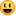59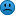7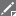19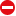2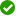2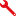2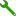6

**Warning! Spoilers may be included in the descriptions or links.Loading Cache Logs...

Current Time:
Last Updated:
Rendered From:Unknown
Coordinates are in the WGS84 datum

Return to the Top of the Page

# Reviewer notes

Use this space to describe your geocache location, container, and how it's hidden to your reviewer. If you've made changes, tell the reviewer what changes you made. The more they know, the easier it is for them to publish your geocache. This note will not be visible to the public when your geocache is published.